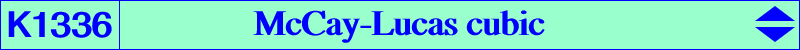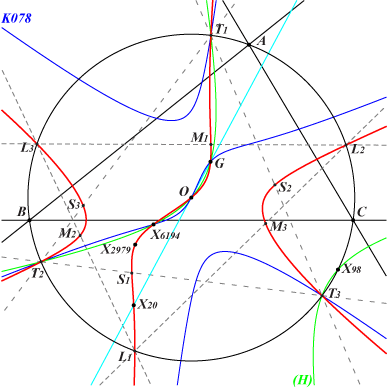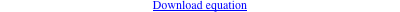too complicated to be written here. Click on the link to download a text file.X(2), X(3), X(20), X(2979), X(6194), X(57450), X(57451) infinite points of the McCay cubic K003 T1, T2, T3 vertices of the CircumTangential triangle, points on K024 L1, L2, L3 vertices of the Lucas triangle, see K007 M1, M2, M3 cevians of O in L1L2L3 S1, S2, S3 pedals of X(2979) in T1T2T3 foci of the conic with center O inscribed in L1L2L3 Geometric properties :K1336 is the McCay cubic of the Lucas triangle hence it is a stelloid. The orthocenter of L1L2L3 is X(2979) and its centroid is X(54041). Hence, the tangents to K1336 at L1, L2, L3 concur at X(2979) and the asymptotes of K1336 are parallel at the radial center X(54041) to those of K003. K078 (the McCay cubic of the Thomson triangle) and K1336 meet at three points on the line at infinity and six finite points which are X(2), X(3), X(6194), T1, T2, T3. These points lie on the rectangular hyperbola (H) passing through X(98), with center X(12042) = midpoint of X(3), X(98). M1, M2, M3, S1, S2, S3 lie on the bicevian conic C(X3, X54041) with respect to the Lucas triangle. The CircumTangential isogonal trransform of K1336 is a central cubic with center O, passing through X(3), X(182), X(3098) and the vertices of the CircumTangential and CircumNormal triangles.